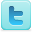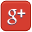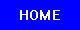Mathematics Learning & Cognition
Science Fair Projects
Ideas and Sample Projects by Grade Level

Primary School - Grades K-3
P=Project   E=Experiment
Find out if playing video games can improve or hurt your math skills. [P]
Interdisciplinary Topic: Intelligence
Elementary School - Grades 4-6
P=Project   E=Experiment
Does playing with Legos every day improve math scores? [E]
Find out if playing the video game "Battlefront 2" affects 5th and 6th grade boys' math abilities after school. [E]
The effect of pre-test exercise on sixth grade students' math test scores. [P] [P]
Does music affect students' math performance? [P] [P]
Interdisciplinary Topic: Intelligence
Middle School - Grades 7-9
P=Project   E=Experiment
Learn if there is a correlation between a parent's attitude towards math and their child's attitude towards math. [E]
Test adults on a math and science tests and decipher the results and their meanings. [E]
Comparing International Math Test Results Using an American Standardized Test [E]
How does human perception of randomness compare to real randomness? [E]
Mathematics: Is There a Gender Difference? [E]
Determine if music would help improve mathematical computations test scores. [E] [P]
Find out which type of music, Jazz or Classical, is better for aiding in mathematical performance. [E]
Determining the Effect of Font Type and Size on Mathematical Computation Ability [E]
The effect of colored vs. white paper on math scores [P] [P]
Does chewing gum during a math test improve test scores? [P]
Interdisciplinary Topic: Intelligence
High School - Grades 10-12
P=Project   E=Experiment
The Effects of Audio Stimulation on Mathematical Test Performance [E]
Math: Are We Born with It? [E]
Are There Distinct Categories of Math Ability? [E]
Does the Language (Number of Phonemes) of Test Questions Affect Arithmetic Calculations and Outcomes? [E]
Test if eating a well-balanced breakfast affects an average teenagers math and puzzle skills. [E]
Interdisciplinary Topic: Intelligence
R=Resource
Science Fair Projects Resources [R]
Citation Guides, Style Manuals, Reference [R]

 Mathematics Projects Math Topics Related Subjects Statistics & Probability Geometry & Trigonometry Applied Mathematics Games & Game Theory Number Theory Mathematics Learning Algebra & Calculus Computer Science Intelligence

 Science Fair Project Guide Home Science Fair Project Types The Scientific Method - How to Experiment The Display Board Topics, Ideas, Sample Projects